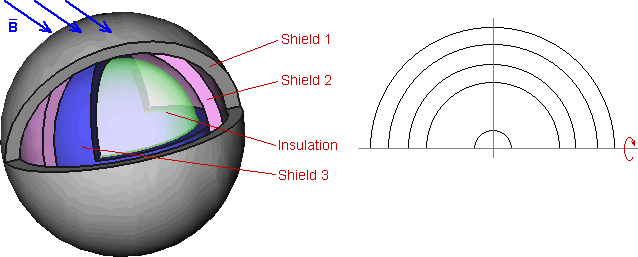QuickField

A new approach to field modelling
:

Main >> Applications >> Sample problems >> Electromagnetic Screen

# Electromagnetic Screen

magnetic shielding simulation, shielding coefficient calculation, temperature dependent magnetic material, multiphysics analysis

Multi-layered shield protects the equipment from the external high-frequency electromagnetic field. The shield should work in wide range of temperatures. Calculate the shielding coefficient as a function of temperature.

Problem Type:
Axisymmetric multiphysics problem of Heat transfer coupled to AC magnetics.

Geometry:Given:
Magnetic permeability of the shields μ = 1;
External field B = 0.03 T;
Frequency f = 4000 Hz;
Temperature range T = -100.. +100 °C;
Device temperature T = 20 °C.
Electrical conductivity of the shields σ - depends on temperature, as shown on the plot below:

The shield conductivity depends on temperature. Calculate the shielding coefficient as a function of temperature.

Solution:
The thermal problem is solved for obtaining the temperature distribution. The result of simulation is transferred to AC magnetics problem, where the magnetic field distribution is calculated. Average flux density is calculated in the 'device' block. Shielding coefficient is then calculated as a ratio of the flux densities on both sides of the screen.
The external magnetic field is defined by the boundary conditions.

Results:
Temperature distribution in the screen:Temperature Flux density in device Shielding coefficient -100 °C 1.0e-8 T 3.3e-7 0 °C 5.2e-8 T 17.3e-7 100 °C 2.8e-7 T 93.3e-7

Magnetic field in the screen:• View simulation report in PDF
• Download simulation files (files may be viewed using any QuickField Edition).